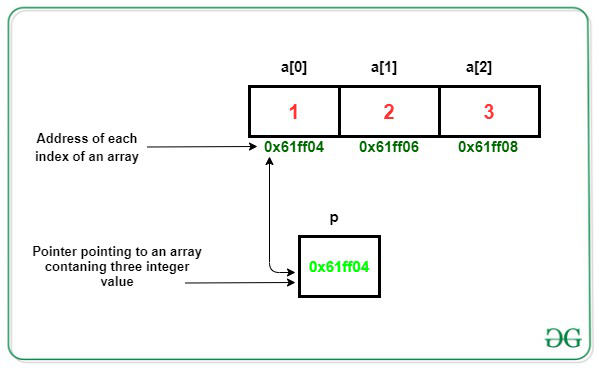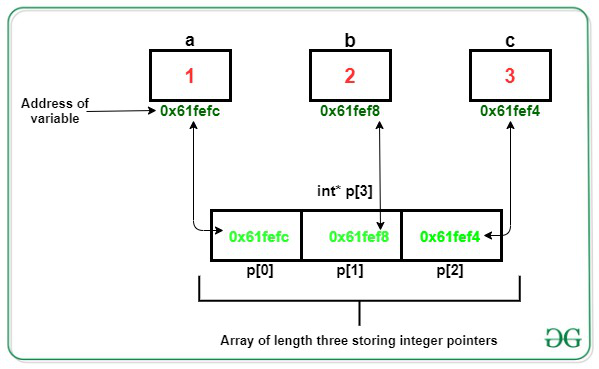# Difference between int (*p) and int* p?

• Difficulty Level : Hard
• Last Updated : 24 Nov, 2020

Pointers store the address of variables or a memory location. Pointers are a symbolic representation of addresses. They enable programs to simulate call-by-reference as well as to create and manipulate dynamic data structures. Its general declaration in C/C++ has the format:

Syntax:

datatype *var_name;

Example:

int *ptr;

In this example “ptr” is a variable name of the pointer that holds address of an integer variable.

In this article, the focus is to differentiate between the two declarations of pointers i.e., int (*p) and int *p.

For int (*p): Here “p” is the variable name of the pointer which can point to an array of three integers.Below is an example to illustrate the use of int (*p):

## C++

 `// C++ program to illustrate the use``// of int (*p)``#include ``using` `namespace` `std;`` ` `// Driver Code``int` `main()``{``    ``// Declaring a pointer to store address``    ``// pointing to an array of size 3``    ``int``(*p);`` ` `    ``// Define an array of size 3``    ``int` `a = { 1, 2, 3 };`` ` `    ``// Store the base address of the``    ``// array in the pointer variable``    ``p = &a;`` ` `    ``// Print the results``    ``for` `(``int` `i = 0; i < 3; i++) {``        ``cout << *(*(p) + i) << ``" "``;``    ``}`` ` `    ``return` `0;``}`

Output:

```1 2 3
```

For int *p: Here “p” is an array of the size 3 which can store integer pointers.Below is an example to illustrate the use of int *p:

## C++

 `// C++ program to illustrate the use``// of int*p``#include ``using` `namespace` `std;`` ` `// Driver Code``int` `main()``{``    ``// Declare an array of size 3 which``    ``// will store integer pointers``    ``int``* p;`` ` `    ``// Integer variables``    ``int` `a = 1, b = 2, c = 3;`` ` `    ``// Store the address of integer``    ``// variable at each index``    ``p = &a;``    ``p = &b;``    ``p = &c;`` ` `    ``// Print the result``    ``for` `(``int` `i = 0; i < 3; i++) {``        ``cout << *p[i] << ``" "``;``    ``}`` ` `    ``return` `0;``}`

Output:

```1 2 3
```

My Personal Notes arrow_drop_up